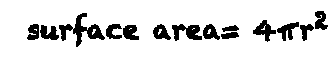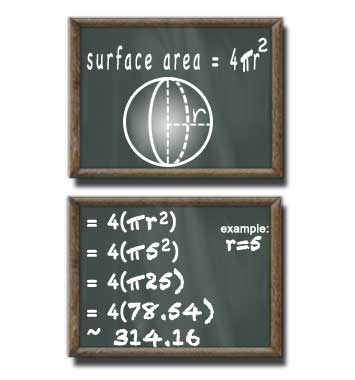# Surface Area of a Sphere Calculator

## Sphere Surface Area

#### For Finding Surface Area of a Sphere

Result:
314.159decimals

A sphere with a radius of 5 units has a surface area of 314.159 square units.

This calculator and more easy to use calculators waiting at www.KylesCalculators.com### Calculating the Surface Area of a Sphere:

Surface Area (denoted 'SA') of a sphere with a known radius (denoted 'r') can be calculated using the formula below:

SA = 4(PI*r2)

In plain english the surface area of a sphere can be calculated by squaring the radius, multiplying the result by PI, and finally multiplying by 4.

You can approximated PI using: 3.14159. If the number you are given for the radius does not have a lot of digits you may use a shorter approximation. If the radius you are given has a lot of digits then you may need to use a longer approximation.

Here is a step-by-step case that illustrates how to find the surface area of a sphere with a radius of 5 meters. We'll use 3.14 to approximate PI because the radius we're given only has one digit.

• V = 4(pi * r2
• = 4(3.14 * 52)
• = 4(3.14 * 25)
• = 4(314.16)
• = 314.16 meters square